# Create Mesh

In the GTD-PO module, the mesh generation is a required step that is performed immediately before simulating. In the Meshing Parameters window, represented in the next Figure, the following options are available:
• Bands Per Octave. Set the different meshes to be generated, according to the bands per octave divisions. If no options are chosen, just a mesh at the lowest simulation frequency is generated. This option is only highlighted when previously a frequency shift or a distance to source sweep are selected.
• On R: the octaves are considered according to the distance to the source. This option only can be set when the source is defined by spherical coordinates.
• On Frequency: the octaves are considered according to the frequency range.
• Number of processors. Sets the number of processors for the meshing process.
• GTD Advanced Parameters: the user can change the curvature angle and the angular margin in order to control how the curved surfaces of the geometry will be partitioned. The contributions are previously evaluated on a parametric internal mesh of the geometry where the curved surfaces are simplified. The resolution of this mesh may be edited according to the below parameters, which are set by default with the recommended values.
• Parametric Steps: accuracy in the curvature evaluation, normalized. Decrease this parameter to get a higher precision in the parametric discretization.
• Curvature angle (deg): maximum curvature considered on targets. It indicates the maximum curvature allowed in the input surfaces for the meshing step. Decrease this parameter to get a higher resolution mesh.
• Angular Margin (deg): maximum deviation allowed around the curvature reference angle. The curvature discretization is achieved by an approximated iterative method, so an angular error around the previous parameter is allowed. Decrease this parameter to increase the precision in the curvature discretization.
• Regular Meshing. The size of the generated elements is selected by the user. Regular meshing is the common algorithm used in other modules such as MOM. It provides a mesh of triangles of similar sizes with a distribution as uniform as possible. It enables the user to set the exact size edge of the triangles to be generated, given in lambda units. However, a huge number of mesh elements may results when the geometry contains large surfaces.
• Multilevel. Use Multilevel to automatically generate the mesh in several sequenced steps. This option is usually more efficient (in terms of runtime) than the common mesh generation option, so it is selected by default. However, there may be minor differences between the meshes obtained with and without the Multilevel mode.
• Edge size. This value is the desired size for the edge of the elements, in wavelengths.
• Curvature Meshing:

Using curvature meshing we get the meshed geometry defined in terms of triangles. This geometry should be very similar to the original geometry. We can force that the meshed geometry be close to the original one using the surface error (in wavelengths). In the mesher we have default values for this magnitude but the user can change it. The mesher tries to minimize the number of triangles of a meshed geometry such that the distance from any point of this geometry to the original one is smaller than the “selected surface error”.

If we have a cube, say the original geometry is defined by six flat quadrangles the meshed geometry is composed by twelve triangles. In this case the distance of any point of the meshed geometry to the original one is null, say both geometries are identical for every frequency.

If an sphere of 1 m of radius at 300 MHz the “selected value” using the defaults values is 0.08*wavelength = 8.0 cm. The mesh is composed by 104 triangles. If you increase the frequency we obtain the same mesh until about 0.7 GHz. Due to topological restriction the number of elements of a mesh of triangle can not increases continuously, say we can not go from 104 triangles to 105 triangles for the sphere (next for the sphere can be 174 triangles).

The Distance error error is other parameter of the meshing by curvature. It only applies to flat facets. The longer edge of the planar surface is split by this parameter. For instance if you set 10 for the cube case you get 12*(102) = 1200 triangles. By default we have 0.01, that is, if this parameter is lower than 1.0 not division of flat facets is consider.

 Regular 1 lambda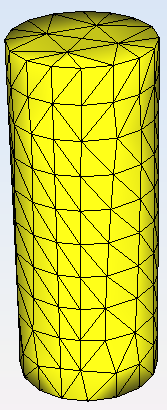0.5 lambda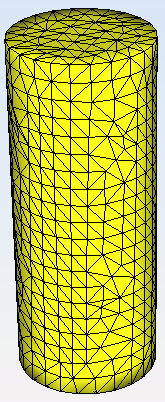0.01 lambda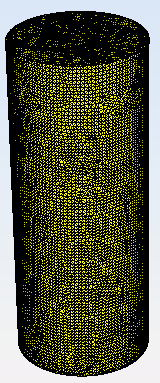Curvature 0.2 surface error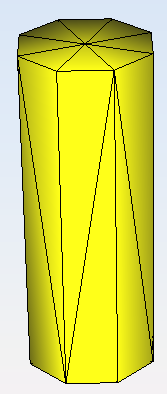0.1 surface error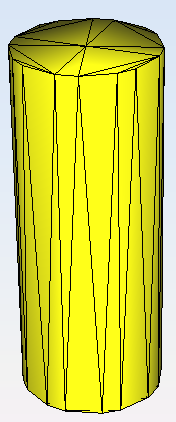0.01 surface error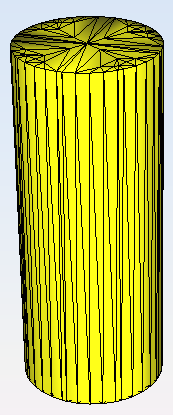The user may select the number of processors used to run the simulation and press the Mesh button to start the meshing process. This process may take a while (depending on the number of processors selected and the computing power of the machine running the simulation).

While running, the status of the meshing process is reported in the process log panel. From this panel, it is possible to abort the running meshing process or save the log to a text file.